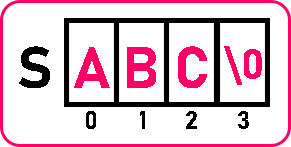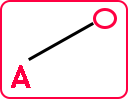# Permutation of Strings in C

## Permutation of Strings in C Language with Examples

In this article, I am going to discuss the Permutation of Strings in C Language with Examples. Please read our previous article where we discussed How to Check if 2 Strings are Anagram in C Language with Examples.

##### Permutation of Strings in C:

In this article, we will see how to find permutations of a string. First of all, we understand what does it mean means by permutations. Then we will see how to find permutations. There is more than one method. In this article, we’ll show you one method.Here we have taken a string ‘ABC’. And we want all permutations with all arrangements of ‘ABC’. The total number of permutations or arrangements we can of string ‘ABC’ is n! which means 3! Which is 6 (3*2*1). So, 6 arrangements are possible for string ‘ABC’. Permutations are:

1. ABC
2. ACB
3. BAC
4. BCA
5. CBA
6. CAB

So, there is a total of six possible arrangements of those alphabets of string ‘ABC’. Now we want all these arrangements, so how to get this arrangement? For that, we will create some procedure or we will create some logic for generating all these permutations. First, let us explain some important things.Here we are making a tree for the permutation of a string. So here the first alphabet is ‘A’.The next alphabet is ‘B’ and the next alphabet is ‘C’. And we got the result ‘ABC’ if we come along this one. So, this is we’re forming a tree. Now from here go back. After that ‘B’ there was only one alphabet. Again, go back to ‘A’. We can go on ‘B’ as well as on ‘C’ so ‘B’ already we have taken. So let us go on ‘C’ then from ‘A’ we have gone on ‘C’ so what is remaining ‘B’ remaining:Here ‘ACB’ will form. We got two different arrangements. So, we can show these arrangements in the form of a tree. After ‘A’, both ‘B’ and ‘C’ are over. So let us go to the next letter ‘B’:The next letter should be either ‘A’ or ‘C’. First, we will take ‘A’ then we will take ‘C’:So, there is another permutation formed that is ‘BAC’. Now go back, only ‘C’ was left as ‘B’ has already been taken.Now we have got ‘BCA’. So let us go back to the main starting point and take the next letter ‘C’:Now we have taken the remaining letter ‘C’. Now, what is remaining under ‘C’? ‘A’ and ‘B’ are remaining. So first we’ll go to ‘A’:Here we got another permutation ‘CAB’. Now go back to ‘C’ and take ‘B’ as ‘A’ has already taken:Now we got our last permutation ‘CBA’. So, we have got the six permutations. Here we did two things Back Tracking and Brute Force.

1. Back Tracking means if we go back and take another possible route in a tree.
2. Brute Force means finding out all possible permutations.

If we have any procedure and, in that procedure, we want to go back and take another route then those procedures can be implemented using Recursion. We have to use Recursion to achieve Back Tracking and with the help of Back Tracking, we are performing Brute Force. Now let’s see the code part:

##### Program for Permutation of Strings in C language by using Loop and Recursion:
#include <stdio.h>
#include <stdlib.h>
void permutation (char s[], int k)
{
static int A = { 0 };
static char Res;
int i;
if (s[k] == ‘\0’)
{
Res[k] = ‘\0’;
printf (“%s\n”, Res);
}
else
{
for (int i = 0; s[i] != ‘\0’; i++)
{
if (A[i] == 0)
{
Res[k] = s[i];
A[i] = 1;
permutation (s, k + 1);
A[i] = 0;
}
}
}
}
int main ()
{
char s[] = “ABC”;
printf (“string is \”%s\”\n”, s);
printf (“Permutations are: \n”);
permutation (s, 0);
return 0;
}

Output:##### Permutation of Strings Code in C language using loop and Recursion:
#include <stdio.h>
#include <stdlib.h>
void swap (char *a, char *b)
{
char temp = *a;
*a = *b;
*b = temp;
}
void permutation (char s[], int l, int h)
{
int i;
if (l == h)
{
printf (“%s\n”, s);
}
else
{
for (i = l; i <= h; i++)
{
swap (&s[l], &s[i]);
permutation (s, l + 1, h);
swap (&s[l], &s[i]);
}
}
}
int main ()
{
char s[] = “ABC”;
printf (“string is \”%s\”\n”, s);
printf (“Permutations are: \n”);
permutation (s, 0, 2);
}

Output: